### Home > CC1 > Chapter 7 > Lesson 7.3.2 > Problem7-100

7-100.
1. For each of the following pairs of fractions, complete the fraction on the right so that the two fractions are equivalent. Using a Giant One might be helpful. Homework Help ✎

1.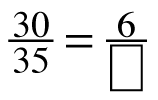2.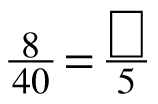3.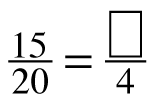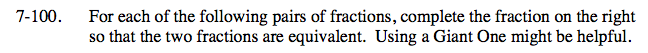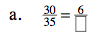The Giant One for this problem could look like this: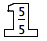Since 35 ÷ 5 = 7, therefore:

$\frac{30}{35}= \frac{6}{7}$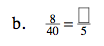Make a Giant One like in part (a).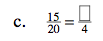The Giant One for this problem could look like this: